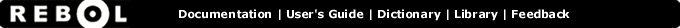# Square-root - Function Summary

## Summary:

Returns the square root of a number.

## Usage:

square-root value

## Arguments:

value - The value argument. (must be: number)

## Description:

Returns the square-root of the number provided. If the number is negative, an error will occur (which you can trap with the TRY function).

 ``` print square-root 4 2.0```

 ``` print square-root 2 1.4142135623731```

## Related:

exp - Raises E (natural number) to the power specified.
log-10 - Returns the base-10 logarithm.
log-2 - Return the base-2 logarithm.
log-e - Returns the base-E (natural number) logarithm.
power - Returns the first number raised to the second number.